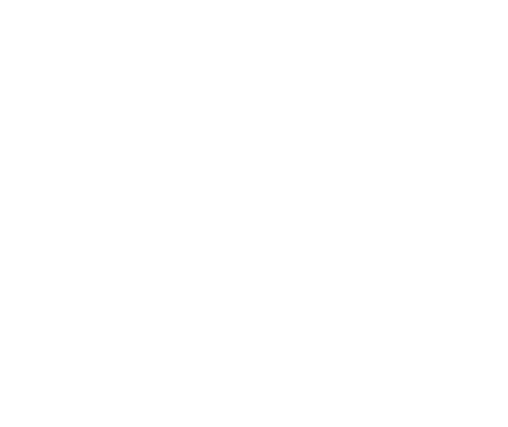# Difference Between Alternating Current and Direct Current

Electricity is the motion of electrons in a conductor and the flow is of two types, namely alternating current (AC) and direct current (DC). Alternating current moves in forward and reverse directions, whereas direct current only moves in a single straight direction. The current which is obtained through dry cells is direct current and the current which runs in electrical wires is alternating current.Let us discuss the difference between alternating and direct current:

### AC v/s DC:

1. The amount of energy carried through the alternating current is more and to the longer distances whereas the amount of energy carried by direct current is to shorter distance only, if traveled long it loses energy.
2. Solar cells , fuel cells and batteries produce direct current and the current which comes from power plants is alternating current.
3. AC can be produced using a special type of generator designed to produce alternating current called an alternator. Direct current is generated from a chemical reaction inside of the battery.
4. AC is the current of magnitude that varies with time, but DC does not vary with time since it has constant magnitude.
5. The passive parameters of alternating current is impedance that is reciprocal of resistance, which is the passive parameter of direct current.
6. The power factor due to fluctuations ranges between 0 & 1 in alternating current where it is only 1 in the case of direct current.
7. The Cause of the direction of flow of electrons in alternating current is rotating magnet along the wire, whereas the cause of direction flow of electrons is steady magnetism along the wire.
8. The frequency of the alternating current is 50 to 60Hz depending on the country and the frequency of the direct current is zero.
9. AC power is discovered by Nikola Tesla and DC power is invented by Thomas Edison in 1800’s.
10. Alternating current can be transferred to a longer distance whereas direct distance can only supply till a mile, which is its major drawback.
11. When there is any issue with the alternating current an electrician can rectify it, but if there is any issue with the DC power , then the battery needs to be changed.
12.  It is difficult to change higher volt DC to low voltage DC current for the domestic use of current and as it moves forward its energy degrades, which is completely opposite in the case of alternating current.
13. The type of waves obtained in AC is sinusoidal, trapezoidal, triangular and square where the shape of DC is pure pulse.

These are the difference between alternating current and direct current for the people who are studying the field of electricity and its behavior. Commercial Electrician Sydney CBD will explain you even more about the electricity and the different types of electricity.

Published
Categorized as News